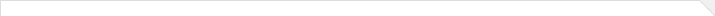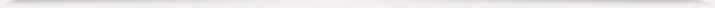报告题目： Bulk universality and quantum unique ergodicity of random band matrices 报 告 人： 杨帆 副教授 报告人所在单位： 清华大学 报告日期： 2023-03-09 报告时间： 13:30-14:30 报告地点： 腾讯会议 ID: 665-541-454 报告摘要： Consider a general class of random band matrices $H$ on the $d$-dimensional lattice of linear size $L$. The entries of $H$ are independent centered complex Gaussian random variables with variances $s_{xy}$, which have a banded profile so that $s_{xy}$ is negligible if $|x-y|$ exceeds the band width $W$. In dimensions $d\ge 7$, assuming that $W\geq L^\delta$ for a small constant $\delta>0$, we prove the deloclaization and quantum unique ergodicity (QUE) of the bulk eigenvectors of $H$. Furthermore, we prove the bulk universality of $H$ under the condition $W \gg L^{95/(d+95)}$. In the talk, I will discuss a new idea for the proof of the bulk universality through QUE, which verifies the conjectured connection between QUE and bulk universality. The proof of QUE is based on a local law for the Green's function of $H$ and a high-order $T$-expansion developed recently. Based on Joint work with Changji Xu, Horng-Tzer Yau and Jun Yin.学术海报.pdf 本年度学院报告总序号： 734